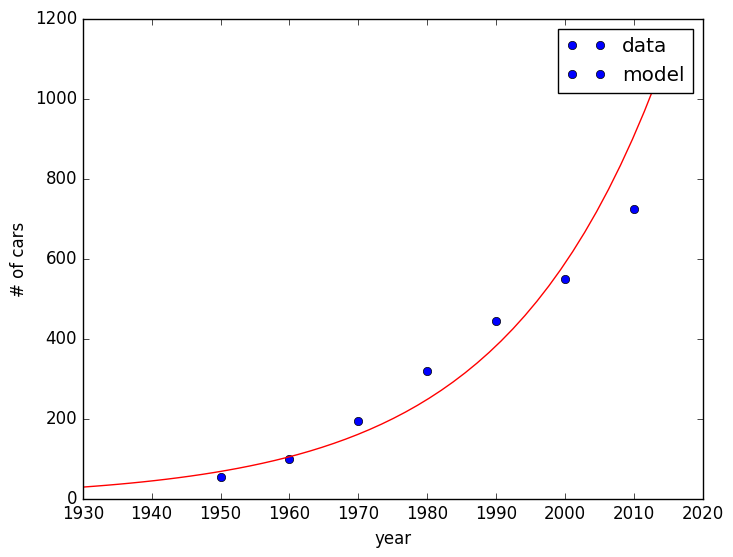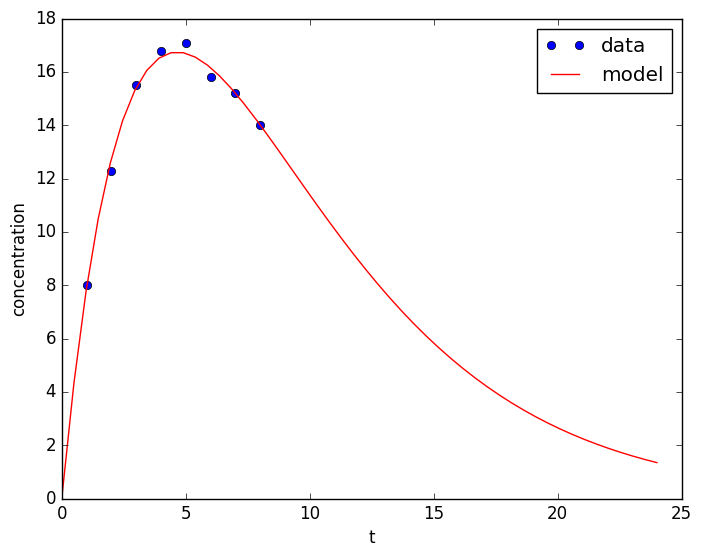# Modeling data with least-squares fits¶

### Exponential model¶

(a) Fit the world population of passenger automobiles to the model $y = c_1 e^{c_2 t}$ by linearizing and doing a least-squares fit for $c_1, c_2$. The number of cars here is given in millions, e.g. there were 53.1 million cars in 1950.

\begin{array}{c|r} year & cars\\ \hline 1950 & 53.1 \\ 1960 & 98.3 \\ 1970 & 193.5 \\ 1980 & 320.3 \\ 1990 & 444.9 \\ 2000 & 548.6 \\ 2010 & 723.6 \end{array}
In :
tdata = [ 1950 1960 1970 1980 1990 2000 2010]

Out:
1×7 Array{Int64,2}:
1950  1960  1970  1980  1990  2000  2010
In :
ydata = [53.1 98.3 193.5 320. 444.9 548.6 723.6]

Out:
1×7 Array{Float64,2}:
53.1  98.3  193.5  320.0  444.9  548.6  723.6
In :
length(t)

Out:
7
In :
A = ones(7,2)
A[:,2] = t
b = log(y)
x = A\b'
c1 = exp(x)
c2 = x

Out:
0.04324088787093803
In :
c1

Out:
1.6248478410492905e-35

(b) Write a Julia function $f(t) = c_1 e^{c_2 t}$ with the numerical values of $c_1, c_2$ and make a plot with the datapoints as dots and the model as a smooth curve.

In :
f(t) = c1 * exp(c2*t)

Out:
f (generic function with 1 method)
In :
using PyPlot
plot(tdata,ydata, "bo")
t = linspace(1930, 2015)
plot(t,f(t), "r-")
legend(("data", "model"))
xlabel("year")
ylabel("# of cars")Out:
PyObject <matplotlib.text.Text object at 0x7f416637d550>

(c) What does the model predict for the worldwide number of cars for 2016? Compare to the actual 2016 value of 1.2 billion cars.

In :
f(2016)

Out:
1174.4624918147235

## Power-law model¶

(a) Fit the height and weight data for males ages 2-11 to a power-law model $w = c_1 h^{c_2}$. The data is from the Centers for Disease Control.

\begin{array}{r|l|l} \text{age (yrs)} & \text{h (m)} & \text{w (kg)} \\ 2 & 0.91 & 13.7 \\ 3 & 0.99 & 15.9 \\ 4 & 1.06 & 18.5 \\ 5 & 1.13 & 21.3 \\ 6 & 1.19 & 23.5 \\ 7 & 1.26 & 27.2 \\ 8 & 1.32 & 32.7 \\ 9 & 1.38 & 36.0 \\ 10 & 1.41 & 38.6 \\ 11 & 1.49 & 43.7 \end{array}
In [ ]:



(b) What weight does the model predict for a 2-meter tall man? What is that in pounds?

In [ ]:



### t e^t model¶

(a) Bloodstream concentration of a drug after hypodermic injection follows the model $y = c_1 t \, e^{c_2 t}$, where $y$ is the drug concentration. Fit the following data to the model, and plot the data and model for $0 \leq t \leq 24$ with dots and curves, respectively.

\begin{array}{r|l} \text{t (hrs)} & \text{concentration (ng/ml)} \\ \hline 1 & 08.0 \\ 2 & 12.3 \\ 3 & 15.5 \\ 4 & 16.8 \\ 5 & 17.1 \\ 6 & 15.8 \\ 7 & 15.2 \\ 8 & 14.0 \end{array}
In :
X = readdlm("blood.asc")
tdata = X[:,1]
ydata = X[:,2]
A = ones(8,2)
A[:,2] = tdata
b = log(ydata./tdata)
x = A\b
c1 = exp(x)
c2 = x

Out:
-0.2151367864153562
In :
c1, c2

Out:
(9.790159861321683,-0.2151367864153562)
In :
f(t) = c1*t.*exp(c2*t)

WARNING: Method definition f(Any) in module Main at In:1 overwritten at In:1.

Out:
f (generic function with 1 method)
In :
plot(tdata, ydata, "bo")
t = linspace(0,24)
plot(t,f(t), "r-")
xlabel("t")
ylabel("concentration")
legend(("data", "model"))Out:
PyObject <matplotlib.legend.Legend object at 0x7f416613bf10>

(b) What is the expected concentration after 12 hours? 18 hrs? 24 hrs?

In :
f(12), f(18), f(24)

Out:
(8.887475142812818,3.6666828137512537,1.3446701506576548)
In [ ]: